# Laboratory Specification Statistics for Microsoft Dynamics AX (Axapta)

## 1. Application Area

Pharma Suite solution includes Analysis Database storing the results of item qualification. This database can be used for statistical observations giving the user an overview about the quality of items and deviations.

Laboratory Specification Statistics (LSS) solution with statistical approach is intended for:

• Calculation of standard deviation for selected sample.
• Creation of statistical charts for visual analysis.

## 2. Analysis Database

Analysis Database is an item oriented one. It includes specification records for each item separated by Analysis Method.

There are 2 kinds of specification records: numerical and alpha. Numerical specification record specifies From-To values (minimax) that an item is to conform. Alpha specification record includes textual description of property and one flag “Conform / Fail”. A specific registration record is created for every batch of material, where the actual measurements are stored.

Only numerical specification is processed in LSS.

## 3. General Demands

LSS supports:

#### Sample Selection

Possibility of sample selection per Item, Vendor, Analysis Method limited by number of entries is supported.

#### Standard Deviation

Standard deviation of selected sample is calculated using:where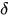- standard deviation,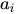- element of sample,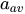- simple average value of sample,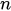- number of sample elements.

#### Quality Control Charts

Quality Control Charts are created based on the results of numeric tests:

• Simple chart. This chart shows sample data “as is” without any mathematical processing.
• Deviation chart. This chart shows the deviation of sample. There are 2 possibilities to select the average value: simple average value of sample and fixed simple average (specified or middle of From-To range).
• CUSUM chart. This chart shows the sum of deviation from sample.

## 4. Dataflow Diagram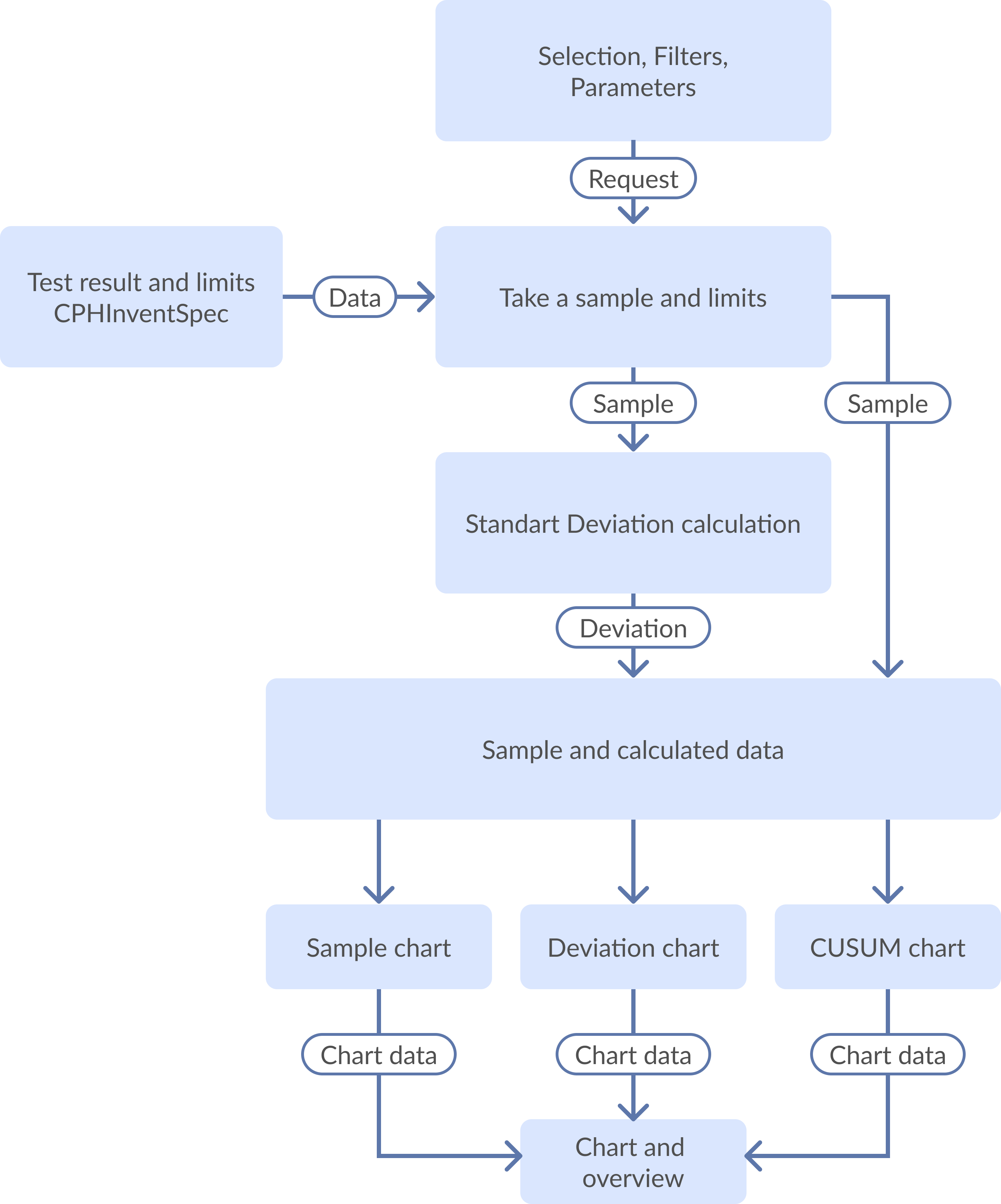Figure 1: Laboratory Specification Statistics dataflow diagram

Here, a sample is build using the data from Analysis Database (Test Results and Limits) and according to the user’s selection (Selection, Filters, and Parameters). Standard deviation is calculated for the sample. Both sample and calculated results are stored in a buffer (sample and calculated data) that is a source for various charts (Sample, Deviation, and CUSUM).